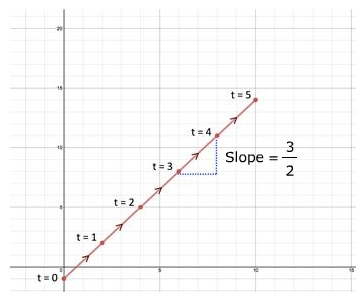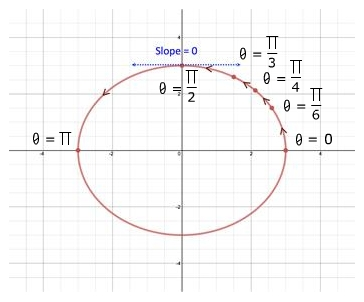# Parametric Equations: Derivatives

Just as with a rectangular equation, the slope and tangent line of a plane curve defined by a set of parametric equations can be determined by calculating the first derivative and the concavity of the curve can be determined with the second derivative.

PARAMETRIC FORM OF THE DERIVATIVE:

If a smooth curve C is given by the continuous functions x = f(t) and y = g(t), then the slope of C and point (x, y) is as follows:

First derivative

$\frac{{d}^{2}y}{d{x}^{2}}=\frac{d}{dx}\left[\frac{dy}{dx}\right]=\frac{\frac{d}{dt}\left[\frac{dy}{dx}\right]}{\frac{dx}{dt}}$           Second derivative

Let's try a couple of examples.

Example 1:Find the slope and concavity of the curve represented by x = 2t and y = 3t - 1 at t = 4 and sketch the curve.

Step 1: Determine the first derivative of both parametric equations with respect to the parameter, $\frac{dx}{dt}$ and $\frac{dy}{dt}$.

First parametric equation

x = 2t Original

$\frac{dx}{dt}=2$ First derivative

Second parametric equation

y = 3t - 1 Original

$\frac{dy}{dt}=3$    First derivative

Step 2: Substitute $\frac{dx}{dt}$ and $\frac{dy}{dt}$ into the parametric derivative equation for the first derivative and calculate the slope, $\frac{dy}{dx}$.

$\frac{dy}{dx}=\frac{\frac{dy}{dt}}{\frac{dx}{dt}}$

$\frac{dy}{dx}=\frac{\frac{dy}{dt}}{\frac{dx}{dt}}$ 1 Deriv. Equation

$\frac{dy}{dx}=\frac{3}{2}=slope$ Substitute

Step 3: Substitute $\frac{dy}{dx}$ and $\frac{dx}{dt}$ into the parametric derivative equation for the second derivative and determine concavity.

$\frac{{d}^{2}y}{d{x}^{2}}=\frac{d}{dx}\left[\frac{dy}{dx}\right]=\frac{\frac{d}{dt}\left[\frac{dy}{dx}\right]}{\frac{dx}{dt}}$

$\frac{{d}^{2}y}{d{x}^{2}}=\frac{d}{dx}\left[\frac{dy}{dx}\right]=\frac{\frac{d}{dt}\left[\frac{dy}{dx}\right]}{\frac{dx}{dt}}$  2 Deriv. Equation

$\frac{{d}^{2}y}{d{x}^{2}}=\frac{\frac{d}{dt}\left[\frac{3}{2}\right]}{2}$ Substitute

$\frac{{d}^{2}y}{d{x}^{2}}=\frac{0}{2}=0$      Take derivative

Step 4: Describe the graph at t = 4.

The slope and concavity of the plane curve at t = 4 is slope $=\frac{3}{2}$ and concavity does not exist.

Parametric Equations: x = 2t , y = 3t - 1

 t 0 1 2 3 4 5 x x = 2t 0 2 4 6 8 10 y y = 3t - 1 -1 2 5 8 11 14Example 2: Find the slope and concavity of the curve represented by and at $\theta =\frac{\pi }{2}$ and sketch the curve.

Step 1: Determine the first derivative of both parametric equations with respect to the parameter, $\frac{dx}{dt}$ and $\frac{dy}{dt}$.

First parametric equation

$x=3\mathrm{cos}\theta$ Original

$\frac{dx}{dt}=-3\mathrm{sin}\theta$      First derivative

Second parametric equation

$y=3\mathrm{sin}\theta$   Original

$\frac{dy}{dt}=3\mathrm{cos}\theta$ First derivative

Step 2: Substitute $\frac{dx}{dt}$ and $\frac{dy}{dt}$ into the parametric derivative equation for the first derivative and calculate the slope, $\frac{dy}{dx}$.

$\frac{dy}{dx}=\frac{\frac{dy}{dt}}{\frac{dx}{dt}}$

$\frac{dy}{dx}=\frac{\frac{dy}{dt}}{\frac{dx}{dt}}$ 1 Deriv. Equation

$\frac{dy}{dx}=\frac{3\mathrm{cos}\theta }{-3\mathrm{sin}\theta }$ Substitute

$\frac{dy}{dx}=-\frac{\mathrm{cos}\theta }{\mathrm{sin}\theta }$ Simplify

$\frac{dy}{dx}=-\mathrm{cot}\theta$ Rewrite

$-\mathrm{cot}\frac{\pi }{2}=0$    Find slope at $\theta =\frac{\pi }{2}$

Step 3: Substitute $\frac{dy}{dx}$ and $\frac{dx}{dt}$ into the parametric derivative equation for the second derivative and determine concavity.

$\frac{{d}^{2}y}{d{x}^{2}}=\frac{d}{dx}\left[\frac{dy}{dx}\right]=\frac{\frac{d}{dt}\left[\frac{dy}{dx}\right]}{\frac{dx}{dt}}$

$\frac{{d}^{2}y}{d{x}^{2}}=\frac{d}{dx}\left[\frac{dy}{dx}\right]=\frac{\frac{d}{dt}\left[\frac{dy}{dx}\right]}{\frac{dx}{dt}}$  2 Deriv. Equation

$\frac{{d}^{2}y}{d{x}^{2}}=\frac{\frac{d}{dt}\left[-\mathrm{cot}\theta \right]}{-3\mathrm{sin}\theta }$ Substitute

$\frac{{d}^{2}y}{d{x}^{2}}=\frac{{\mathrm{csc}}^{2}\theta }{-3\mathrm{sin}\theta }$   Take deriv.

Find concavity at $\theta =\frac{\pi }{2}$

$\frac{{\mathrm{csc}}^{2}\theta }{-3\mathrm{sin}\theta }=\frac{{\mathrm{csc}}^{2}\frac{\pi }{2}}{-3\mathrm{sin}\frac{\pi }{2}}=-\frac{1}{3}$ Concave down

Step 4: Describe the graph at $t=\frac{\pi }{2}$.

The slope and concavity of the plane curve at $t=\frac{\pi }{2}$ is slope = 0 and concavity is down.

Parametric Equations: $x=3\mathrm{cos}\theta$ , $y=3\mathrm{sin}\theta$

 θ 0 $\frac{\pi }{6}$ $\frac{\pi }{4}$ $\frac{\pi }{3}$ $\frac{\pi }{2}$ π x 3 $\frac{3\sqrt{3}}{2}$ $\frac{3\sqrt{2}}{2}$ $\frac{3}{2}$ 0 -3 y 0 $\frac{3}{2}$ $\frac{3\sqrt{2}}{2}$ $\frac{3\sqrt{3}}{2}$ 3 0Related Links: Math algebra Inverse Functions: Graphs Inverse Functions: One to One Pre Calculus

To link to this Parametric Equations: Derivatives page, copy the following code to your site: